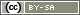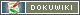# random_items()

`string random_items(array|string basis, [int number])`

Randomly selects number of items from a list of items or from all items in a question.

• basis
A list of items (array or comma-separated) or a question ID
• number
Number of items that should be drawn. If the second parameter is omitted, the items will be returned in a random order.

Note: As a rule, enabling item rotation directly in the question (Items' order → “Shuffle/rotate randomly”) is preferred over using the function `random_items()`. This function only provides real added value if the same random selection is needed again in a later question, or if not all of the items should be displayed.

## Examples

It is assumed that questions AB01 and AB02 have the same items. The following PHP code shows the items in question AB01 in a random order – and the items in question AB02 in the same order.

```\$items = random_items('AB01');
question('AB01', \$items);
question('AB02', \$items);```

Tip: If questions AB01 and AB02 should be asked on different pages, use `registerVariable(\$items)` to make the variable `\$items` available for use on future pages.

In the following example, 3 items are randomly selected from items 1,2,3,4,9 and 9, and questions AB01 and AB02 are only shown with these items.

```\$items = random_items('1-4,8,9', 3);
question('AB01', \$items);
question('AB02', \$items);```

The following PHP code shows 3 of the items from question AB01 at random.

```\$items = random_items('AB01', 3);
question('AB01', \$items);```

The same result can be also obtained, however, with a corresponding parameter in the command `question()`.

`question('AB01', 'random=3');`